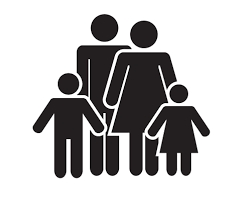# Father 7470

The father is eight years older than three times the son's age. In 20 years, the father will be twice as old as his son. How many years is a father, and how many are a son?

o =  44
s =  12

### Step-by-step explanation:

o=8+3s
o+20 = 2·(s+20)

o=8+3·s
o+20 = 2·(s+20)

o-3s = 8
o-2s = 20

Row 2 - Row 1 → Row 2
o-3s = 8
s = 12

s = 12/1 = 12
o = 8+3s = 8+3 · 12 = 44

o = 44
s = 12

Our linear equations calculator calculates it.Did you find an error or inaccuracy? Feel free to write us. Thank you!

Tips for related online calculators
Do you have a linear equation or system of equations and looking for its solution? Or do you have a quadratic equation?
Do you want to convert time units like minutes to seconds?